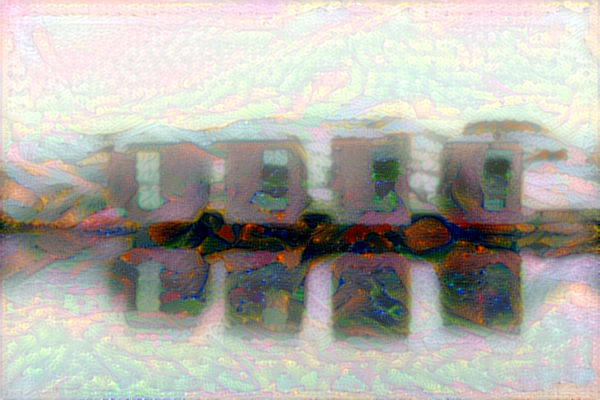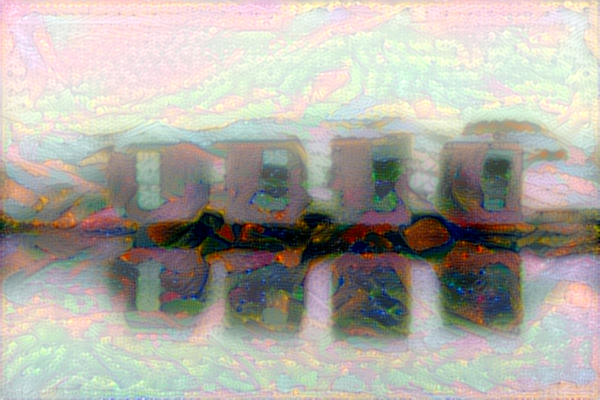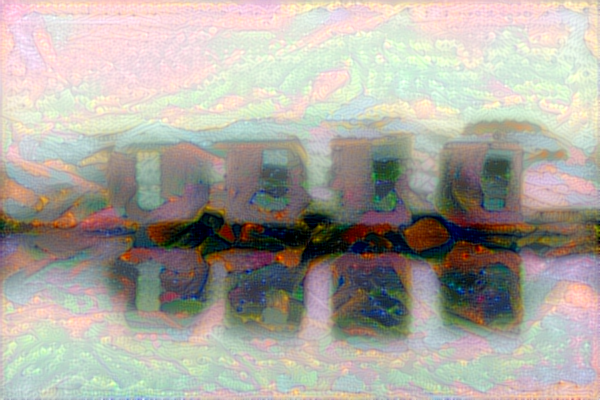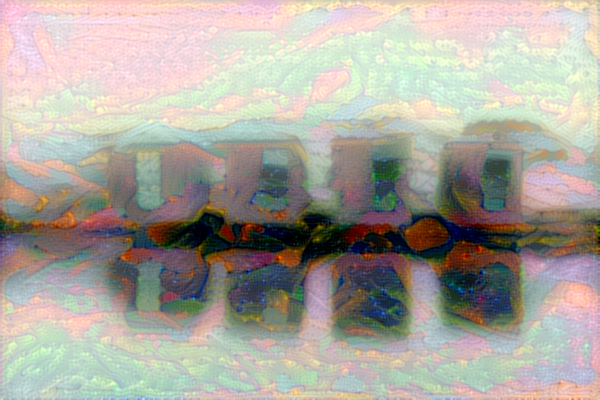# Keras Neural Style Transfer

pip install --upgrade pillow

Gauguin is our style reference.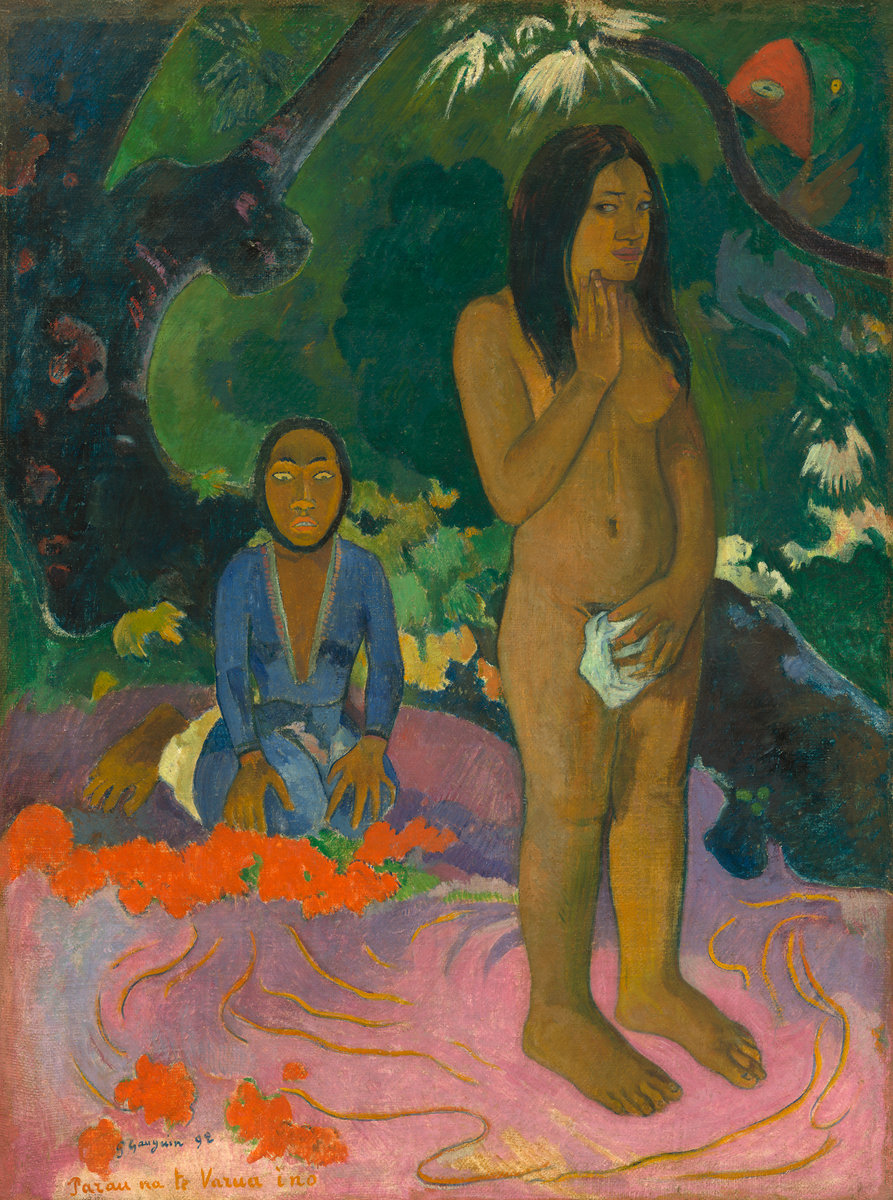Gauguin, Parau na te Varua ino (Words of the Devil), 1892

And we want to Gauguinize the swedish Nordiska Akvarellmuseet

import PIL.Image
from keras.preprocessing.image import load_img, save_img, img_to_array
import numpy as np
from scipy.optimize import fmin_l_bfgs_b, minimize
import time
from keras.applications import vgg19
from keras import backend as K
base_image_path = Image↩
style_reference_image_path = Image↩

result_prefix = '/results/'
iterations = 5

# these are the weights of the different loss components
total_variation_weight = 1.0
style_weight = 1.2
content_weight = 0.025

# dimensions of the generated picture.
img_nrows = 400
img_ncols = int(width * img_nrows / height)
0.1s
def preprocess_image(image_path):
img = img_to_array(img)
img = np.expand_dims(img, axis=0)
img = vgg19.preprocess_input(img)
return img

# util function to convert a tensor into a valid image
def deprocess_image(x):
if K.image_data_format() == 'channels_first':
x = x.reshape((3, img_nrows, img_ncols))
x = x.transpose((1, 2, 0))
else:
x = x.reshape((img_nrows, img_ncols, 3))
# Remove zero-center by mean pixel
x[:, :, 0] += 103.939
x[:, :, 1] += 116.779
x[:, :, 2] += 123.68
# 'BGR'->'RGB'
x = x[:, :, ::-1]
x = np.clip(x, 0, 255).astype('uint8')
return x

# the gram matrix of an image tensor (feature-wise outer product)

def gram_matrix(x):
assert K.ndim(x) == 3
if K.image_data_format() == 'channels_first':
features = K.batch_flatten(x)
else:
features = K.batch_flatten(K.permute_dimensions(x, (2, 0, 1)))
gram = K.dot(features, K.transpose(features))
return gram

# the "style loss" is designed to maintain
# the style of the reference image in the generated image.
# It is based on the gram matrices (which capture style) of
# feature maps from the style reference image
# and from the generated image

def style_loss(style, combination):
assert K.ndim(style) == 3
assert K.ndim(combination) == 3
S = gram_matrix(style)
C = gram_matrix(combination)
channels = 3
size = img_nrows * img_ncols
return K.sum(K.square(S - C)) / (4. * (channels ** 2) * (size ** 2))

# an auxiliary loss function
# designed to maintain the "content" of the
# base image in the generated image

def content_loss(base, combination):
return K.sum(K.square(combination - base))

# the 3rd loss function, total variation loss,
# designed to keep the generated image locally coherent

def total_variation_loss(x):
assert K.ndim(x) == 4
if K.image_data_format() == 'channels_first':
a = K.square(x[:, :, :img_nrows - 1, :img_ncols - 1] - x[:, :, 1:, :img_ncols - 1])
b = K.square(x[:, :, :img_nrows - 1, :img_ncols - 1] - x[:, :, :img_nrows - 1, 1:])
else:
a = K.square(x[:, :img_nrows - 1, :img_ncols - 1, :] - x[:, 1:, :img_ncols - 1, :])
b = K.square(x[:, :img_nrows - 1, :img_ncols - 1, :] - x[:, :img_nrows - 1, 1:, :])
return K.sum(K.pow(a + b, 1.25))

if K.image_data_format() == 'channels_first':
x = x.reshape((1, 3, img_nrows, img_ncols))
else:
x = x.reshape((1, img_nrows, img_ncols, 3))
# f_outputs is defined below
outs = f_outputs([x])
loss_value = outs
if len(outs[1:]) == 1:
else:
return loss_value, grad_values
6.0s
# get tensor representations of our images
base_image = K.variable(preprocess_image(base_image_path))

style_reference_image = K.variable(preprocess_image(style_reference_image_path))

# this will contain our generated image
print(K.image_data_format())

if K.image_data_format() == 'channels_first':
combination_image = K.placeholder((1, 3, img_nrows, img_ncols))
else:
combination_image = K.placeholder((1, img_nrows, img_ncols, 3))

# combine the 3 images into a single Keras tensor
input_tensor = K.concatenate([base_image,
style_reference_image,
combination_image], axis=0)

# build the VGG19 network with our 3 images as input
# the model will be loaded with pre-trained ImageNet weights
model = vgg19.VGG19(input_tensor=input_tensor,
weights='imagenet', include_top=False)

# get the symbolic outputs of each "key" layer (we gave them unique names).
outputs_dict = dict([(layer.name, layer.output) for layer in model.layers])

# combine loss functions into a single scalar
loss = K.variable(0.)
layer_features = outputs_dict['block5_conv2']
base_image_features = layer_features[0, :, :, :]
combination_features = layer_features[2, :, :, :]
loss += content_weight * content_loss(base_image_features,
combination_features)

# layers
feature_layers = ['block1_conv1', 'block2_conv1',
'block3_conv1', 'block4_conv1',
'block5_conv1']

for layer_name in feature_layers:
layer_features = outputs_dict[layer_name]
style_reference_features = layer_features[1, :, :, :]
combination_features = layer_features[2, :, :, :]
sl = style_loss(style_reference_features, combination_features)
loss += (style_weight / len(feature_layers)) * sl

loss += total_variation_weight * total_variation_loss(combination_image)

# get the gradients of the generated image wrt the loss

outputs = [loss]
else:

f_outputs = K.function([combination_image], outputs)
class Evaluator(object):

def __init__(self):
self.loss_value = None

def loss(self, x):
assert self.loss_value is None
self.loss_value = loss_value
return self.loss_value

assert self.loss_value is not None
self.loss_value = None

evaluator = Evaluator()
x = preprocess_image(base_image_path)
for i in range(iterations):
print('Start of iteration', i)
start_time = time.time()

# fmin_l_bfgs_b
x, min_val, info = fmin_l_bfgs_b(evaluator.loss,
x.flatten(),
maxfun=50)

print('Current loss value:', min_val)
# save current generated image
img = deprocess_image(x.copy())
fname = result_prefix + '_at_iteration_%d.png' % i
save_img(fname, img)
end_time = time.time()
print('Image saved as', fname)
print('Iteration %d completed in %ds' % (i, end_time - start_time))